# Energy dissipated in the resistors in a 2 mesh RC circuit

Eduardo Leon

## Homework Statement

Hi mates, I have problems solving the third part of this exercise, I've already done all the previous calculations.

Given the following circuit, where the switch S is open, the power supply = 50 volts and:
• The initial charge in the C capacitor: QC = 0 coulombs
• The initial charge in the 2C capacitor: Q2C = 20^-6 coulombs
Calculate (with real instruments):
1. Switch⇒I find the current passing by the amperemeter (IA) and the voltage across the voltmeter VV After a long time t (t>5 time constants). (Done).
2. Switch⇒II find the voltage across the voltmeter VV passed 10 milliseconds. (Done).
3. Switch⇒III next get the final reading of the voltmeter VV at a given time t (t >0 ∧ t< 5 time constants) and the energy dissipated in the resistors till that instant.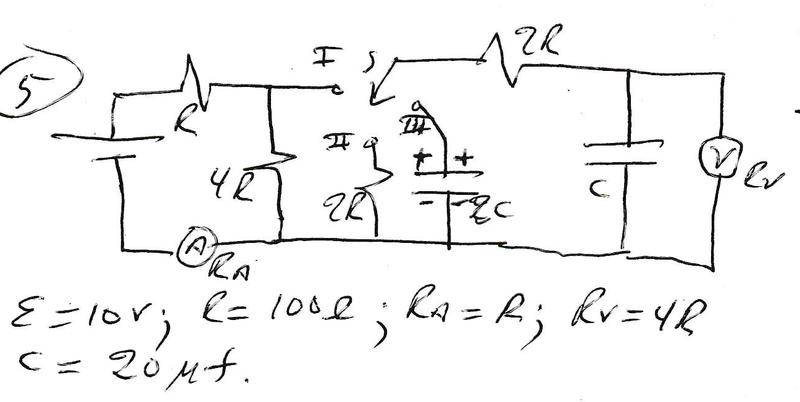## Homework Equations

• Ohm's law: R=ΔV/i
• Capacitance: C=q/ΔV
• Kirchoff's laws: Σi=0 ∧ ΣΔV=0
• Energy gived by the battery until an instant t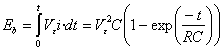• Energy dissipated in the resistor until an instant t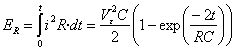• Energy stored in the capacitor until an instant t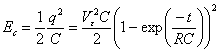## The Attempt at a Solution

As stated before, I've done the first and the second parts.

For the first:
•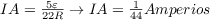Amps*
•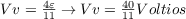Volts*
For the second:
•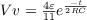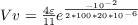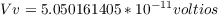Volts*
For the third:
Well, here is where i get lost, because I dont't know how to study and analize the circuit formed at this point, I've only seen discharging RC circuits whis a single current, one resistor and one capacitor due those elements could be joined into their equivalent ones, but here, I get 2 resistors, 2 capacitors and 2 meshes, like this one.I know that the initial charges at this point are:

In order to get the initial charge in the C capacitor I used the voltage obtained in the voltmeter in the second part at the 10 milliseconds, because are connected parallel. So, multiplying that voltage times the capacitance, we get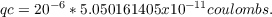The initial charge in the 2C capacitor stills equal, since its an ideal capacitor, so is the same: Q2C = 20^-6 coulombs

#### Attachments

Last edited by a moderator: